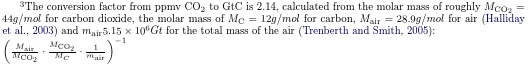# Conversion factor from ppmv CO₂ to Gt C

I just spent half an hour on finding the references for this, so I can spend 5 minutes providing it for others on the web.The conversion factor from ppmv CO$$_2$$ to GtC is 2.14, calculated from the molar mass of roughly $$M_{\text{CO}_{2}} = 44 g/mol$$ for carbon dioxide, the molar mass of $$M_{\text{C}} = 12 g/mol$$ for carbon, $$M_{\text{air}} = 28.9 g/mol$$ for air (Halliday et al., 2003) and $$m_{\text{air}} 5.15 \times 10^{6} Gt$$ for the total mass of the air (Trenberth and Smith, 2005): $$\left ( \frac{M_{\text{air}}}{M_{\text{CO}_{2}}} · \frac{M_{\text{CO}_{2}}}{M_{C}} · \frac{1}{m_{\text{air}}} \right )^{-1}$$

(let ((Mco2 44.0) ; g / mol
(Mair 28.9) ; g / mol
(Mc 12.0) ; g / mol
(mair 5.15)) ; 1,000,000 Gt
(/ 1
(* (/ Mair Mco2)
(/ (/ Mco2 Mc) mair))))


Halliday et al., 2003: Halliday, D., Resnick, R., Walker, J., and Koch, S. (2003). Physik. Wiley.

Trenberth and Smith, 2005: Trenberth, K. E. and Smith, L. (2005). The mass of the atmosphere: A constraint on global analyses. Journal of Climate, 18(6):864–875.

PS: GtC Gigaton Carbon = PgC Petagram Carbon; ppmv CO₂ = parts per million (in volume) carbon dioxide in air.

AnhangGröße
conversion-factor-ppmv-co2-to-gtc.png18.27 KB

Use Node:

⚙ Babcom is trying to load the comments ⚙

This textbox will disappear when the comments have been loaded.

If the box below shows an error-page, you need to install Freenet with the Sone-Plugin or set the node-path to your freenet node and click the Reload Comments button (or return).

If you see something like Invalid key: java.net.MalformedURLException: There is no @ in that URI! (Sone/search.html), you need to setup Sone and the Web of Trust

If you had Javascript enabled, you would see comments for this page instead of the Sone page of the sites author.

Note: To make a comment which isn’t a reply visible to others here, include a link to this site somewhere in the text of your comment. It will then show up here. To ensure that I get notified of your comment, also include my Sone-ID.

Link to this site and my Sone ID:  sone://6~ZDYdvAgMoUfG6M5Kwi7SQqyS-gTcyFeaNN1Pf3FvY

This spam-resistant comment-field is made with babcom.Willkommen im Weltenwald!
((λ()'Dr.ArneBab))